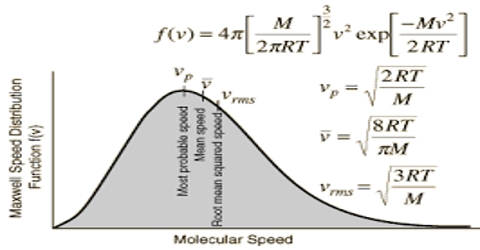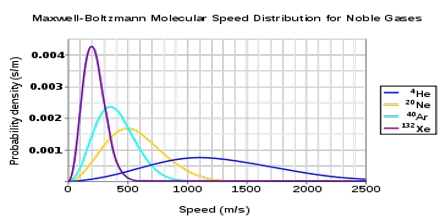# Distribution of Velocities

Distribution of Velocities

A result of the chaotic movement of molecules in the gaseous state is that in addition to their collisions with the walls of the container they collide with each other. Collisions with other molecules continually change the speed of a particular molecule. A particular molecule may move along a given direction until it hits another, to which it loses a pan of its kinetic energy. In the next collision with a third particle it may gain some kinetic energy and change its direction of movement. The path of each molecule is completely haphazard. The exchange of kinetic energy between colliding molecules goes on continually. The total kinetic energy of a given mass of gas, however, remains constant as long as the temperature remains the same. The total kinetic energy of a gas is made up of the contributions of all the molecules, each of which may he moving with different speed. At any instant some of the molecules may he moving with very high velocities.  Some may have very low velocities (may even be standing still) while the majority will have velocities near the average.

Since the velocities are determined by chance collisions and a large number of molecules are involved, Maxwell and & Boltzmann applied the laws of probability to the situation obtaining in a gas and derived the following expression for the distribution of velocities among gas molecules:

dn/n = 4π (M/2πRT)3/2 x e– (mc2/2RT) x c2dc

where M is the molecular mass, c is the velocity, n is the total number of molecules, dn/n is the fraction of molecules having velocity between c and c+dc (dc being infinitesimally small increment). T as the absolute temperature and R is the universal gas constant. This is known as the Maxwell-Boltzmann distribution equation. Figure is a graph representing equation (1). Such a graph is known as Maxwell-Boltzmann distribution curve.The values of dn/n have been calculated from the right side of the equation (1) by putting different values of c. Each point in the curve tells what fraction of the molecules velocity has specified by the value of the abscissa at the point.

It is evident from the distribution equation that the value of dn/n is profoundly influenced by temperature. The distribution at the higher temperature takes the form shown in Figure. At the higher temperature the molecules have higher average kinetic energy, i.e., higher velocity than at the lower temperature. Thus, temperature serves to measure the average kinetic energy.